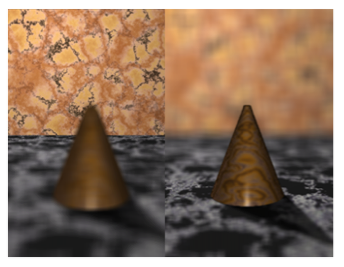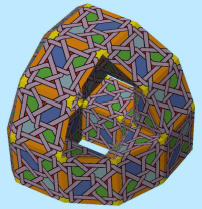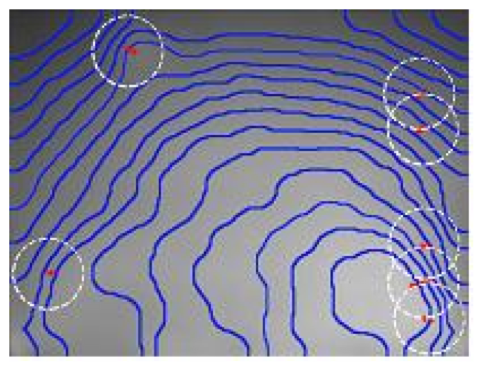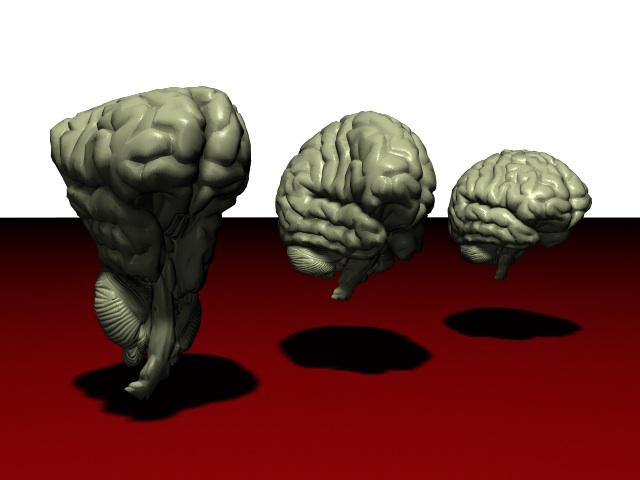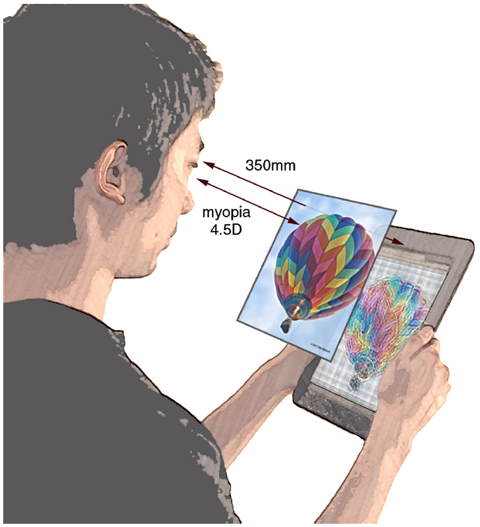# Computer Graphics

## An Energy-Driven Approach to Linkage Unfolding

### Abstract

We present a new algorithm for unfolding planar polygonal linkages without self-intersection based on following the gradient flow of a "repulsive" energy function. This algorithm has several advantages over previous methods. (1) The output motion is represented explicitly and exactly as a piecewise-linear curve in angle space. As a consequence, an exact snapshot of the linkage at any time can be extracted from the output in strongly polynomial time (on a real RAM supporting arithmetic, `sin` and `arcsin`). (2) Each linear step of the motion can be computed exactly in O(n2) time on a real RAM where n is the number of vertices. (3) We explicitly bound the number of linear steps (and hence running time) as a polynomial in n and the ratio between the maximum edge length and the initial minimum distance between a vertex and an edge. (4) Our method is practical and easy to implement. We provide a publicly accessible Java applet that implements the algorithm.
Best paper award at SoCG 2004.

### Citation

Jason H. Cantarella, Eric D. Demaine, Hayley N. Iben, and James F. O'Brien. "An Energy-Driven Approach to Linkage Unfolding". In Proceedings of the 20th Annual Symposium on Computational Geometry, June 2004.

### Supplemental Material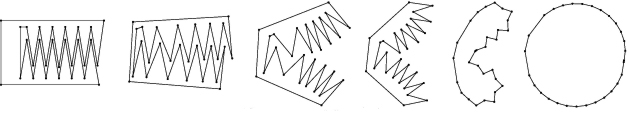Teeth with energy method.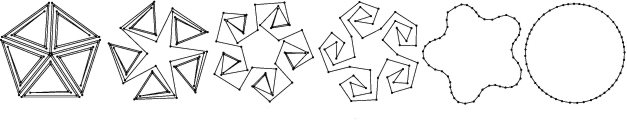Tree with energy method.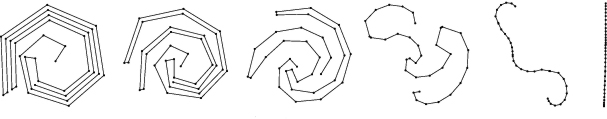A spiral open chain.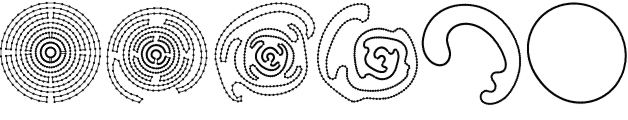Tentacle opening.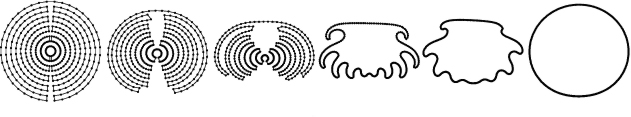Spider shape opening.

#### Demonstration Applet

The Unfold Applet will appear below in a Java enabled browser.